﻿ 工件图像匹配的局部敏感哈希应用改进 An Improvement on Workpiece Image Matching via Locality Sensitive Hashing

Computer Science and Application
Vol.08 No.05(2018), Article ID:25196,10 pages
10.12677/CSA.2018.85090

An Improvement on Workpiece Image Matching via Locality Sensitive Hashing

Xiuli Shao, Zihao Dong, Zihan Li, Jingya Yang

College of Computer and Control Engineering, Nankai University, Tianjin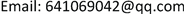Received: May 9th, 2018; accepted: May 23rd, 2018; published: May 30th, 2018ABSTRACT

The extraction and matching of workpiece contour is one of the most important steps in robot sorting. The traditional contour matching algorithm uses Hausdorff distance, with considerable amount of calculation and difficulty in determining the position and angle of the workpiece. Based on the template matching, the image feature vector is used to match the image in this paper, which reduces the computation and solves the problem of image recognition. We also improve the traditional linear search algorithm by using the Locality Sensitive Hashing algorithm. Moreover, the hash algorithm is improved to reduce the storage space. In the experiment, the algorithm can effectively ignore the problems caused by the translation, zoom and rotation of workpiece during image recognition. What’s more, it can improve the efficiency and accuracy of image search to a certain extent.

Keywords:Workpiece Matching, Locality Sensitive Hashing, And/Or Operation1. 概述

2. 基于局部敏感哈希存储提取的工件图像特征

Hash桶的性质：如果两个输入的元素经过映射后所在的桶不同，那么这两个元素一定不相同。而如果映射后在同一桶中，则说明两元素有可能相同但并不是一定相同。

1) 若 $d\left(x,y\right)\le {d}_{1}$ ，则两元素被映射到同一个桶中的概率，即 $h\left(x\right)=h\left(y\right)$ 的概率至少为p1

2) 若 $d\left(x,y\right)\le {d}_{2}$ ，则两元素被映射到同一个桶中的概率，即 $h\left(x\right)=h\left(y\right)$ 的概率至多为p2

3. 工件特征的哈希表的生成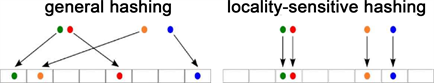Figure 1. Comparison of local sensitive Hash and traditional Hash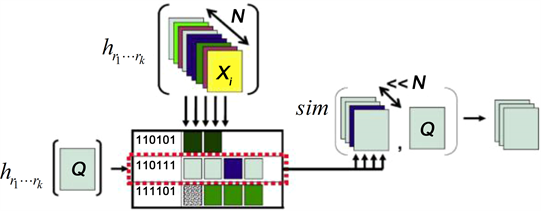Figure 2. The schematic diagram of LSH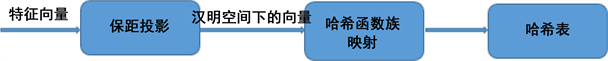Figure 3. The generation process of Hash table

3.1. 保距投影

$V\left(p\right)=Unar{y}_{c}\left({x}_{1}\right)Unar{y}_{c}\left({x}_{2}\right)Unar{y}_{c}\left({x}_{3}\right)\cdots Unar{y}_{c}\left({x}_{n}\right)$ (1)

3.2. 哈希函数和哈希表的形成

4. 工件的图像匹配Figure 4. The flow chart of image matching

4.1. 与或操作

1) 与操作

2) 或操作

4.2. 桶内排序

1) jaccard距离

jaccard相似度是指两集合交集的元素个数比上两集合并集的元素个数，具体公式如下：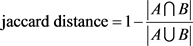(2)

2) 夹角距离

3) 欧几里得距离

$A=\left[{x}_{1},{x}_{2},{x}_{3},\cdots ,{x}_{n}\right]$

$B=\left[{y}_{1},{y}_{2},{y}_{3},\cdots ,{y}_{n}\right]$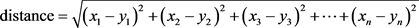(3)

5. 主要函数的实现

1) lsh函数，用户调用该函数，并传入哈希表中表的个数l和桶的个数k以及原始数据空间的维数d和原始数据varagin便可以得到映射过后的哈希表

2) lshfunc函数，创造随机的哈希函数族，在lsh函数中被调用。

3) lshprep函数，创建l个哈希表，被给每个哈希表设置其哈希函数。

4) lshins函数，在lsh函数中被调用，将原始数据通过上述lshfunc函数获得的哈希函数族映射到哈希表中。且为了减少占用的内存，这里表中并不存储特征向量，只存储特征向量在向量库中的索引值。

5) lshlookup函数是局部敏感哈希的查询代码，其中传入参数temp_T是当前工件的特征向量，Patches是模板特征库，T1是已经建立好的哈希表，函数可以返回与temp_T投影在同一桶中的特征向量的个数，以及其在特征库中的索引。

6) lsh (l, k, d, x, varargin)函数调用lshfunc生成函数族，然后使用lshprep创建哈希表，调用lshins进行投影，其核心代码较为简单，这里不做赘述。

7) lshfunc函数，按照用户传入的桶的个数随机选取几个维度，给这些维度设置一个阀值t，hash函数为x(d) ≥ t。

6. 实验测试

6.1. 图像匹配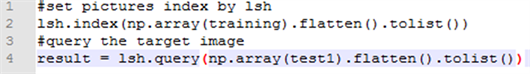LSH检索器得出的检索结果如图8所示。即选择图7仿射变换后的工件轮廓为测试集，通过LSH算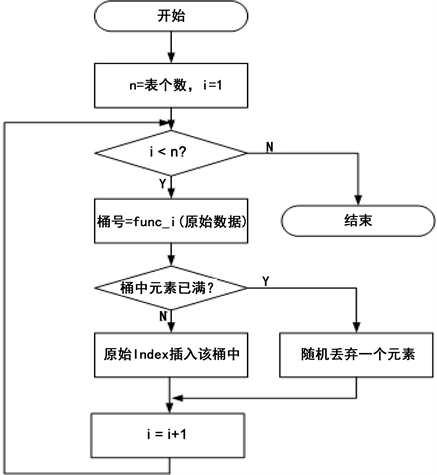Figure 5. The flow chart of lshins function.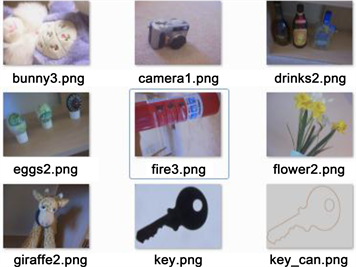Figure 6. The workpiece dataset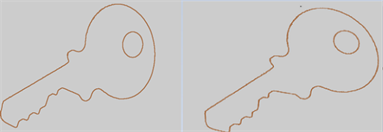Figure 7. The Edge of the original workpiece and the one after affine transformation.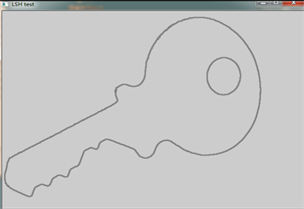Figure 8. The retrieval result of LSH

$r=\frac{{\sum }_{i}\left[\left(x\left(i\right)-mx\right)\ast \left(y\left(i\right)-my\right)\right]}{\sqrt{{\sum }_{i}{\left(x\left(i\right)-mx\right)}^{2}}\sqrt{{\sum }_{i}{\left(y\left(i\right)-my\right)}^{2}}}$ (4)

6.2. 特征存储

7. 实验结果

8. 结论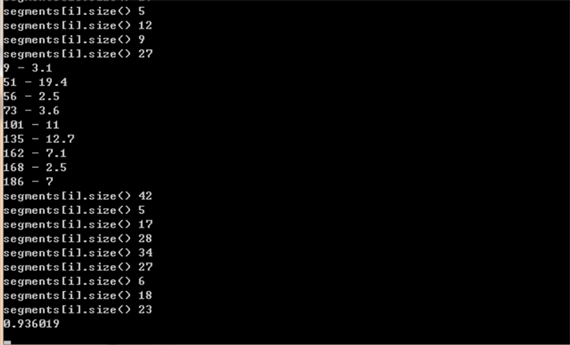Figure 9. The correlation of real workpiece edge map and the one retrieved by LSH methodFigure 10. The overall flow chart of this retrieval system

16ZXHLGX00250、15ZXHLGX00360、15ZXHLGX00380、15ZXDSGX00090。

An Improvement on Workpiece Image Matching via Locality Sensitive Hashing[J]. 计算机科学与应用, 2018, 08(05): 809-818. https://doi.org/10.12677/CSA.2018.85090

1. 1. Bourret, P. and Cabon, B. (1995) Neural Approach for Satellite Image Registration and Pairing Segmented Areas. SPIE, 2576, 22.

2. 2. 梁勇, 祝明波, 杨汝良. 一种基于Hausdorff度量的多传感器图像配准方法[J]. 遥感技术与应用, 2006, 21(5): 473-476.

3. 3. 张学军. 基于支持向量机的红外图像匹配研究[D]. 华中科技大学, 2007.

4. 4. 刘健庄, 谢维信, 高新波, 等. 基于Hausdorff距离和遗传算法的物体匹配方法[J]. 电子学报, 1996.

5. 5. 贺雅琴. 自动物料分拣机器人系统的关键技术研究[D]. 华南理工大学, 2011.

6. 6. 凌康, 武港山. 基于位置敏感哈希的相似性搜索技术研究[D]. 南京大学, 2012.